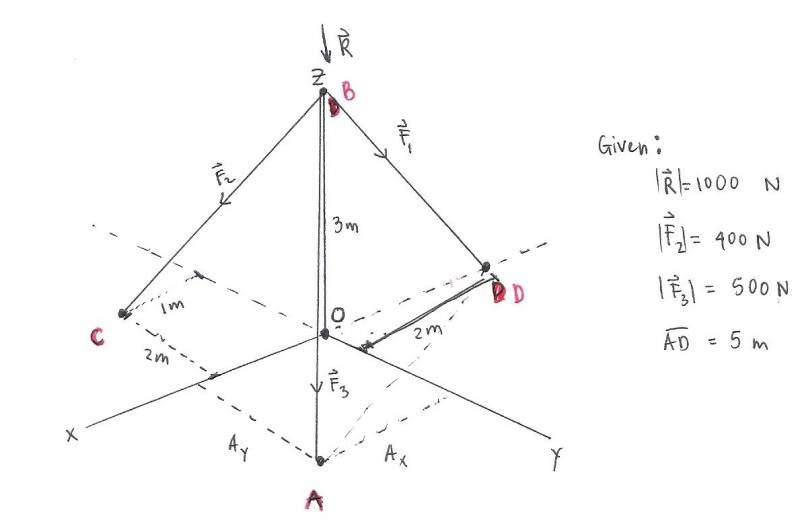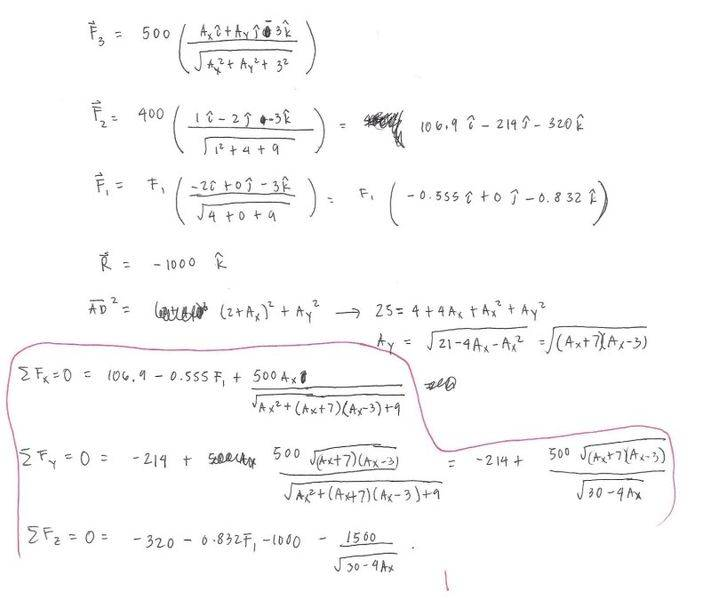# Resolving Forces in 3D - Statics

keximaze
1. Three guy wires hold a pole BO as shown. The resultant R of the forces due to the three wires has a magnitude of 1000-N and is directed on the pole BO. Force F2 and F3 have a magnitude of 400-N and 500-N, respectively. The distance from point A to point D is 5-m. Find the tension in cable BD.## Homework Equations

Summation of all the forces = 0
F1 + F2 + F3 + R = 0
F = F*(unit vector)

## The Attempt at a Solution

On the solution below, I first established vectors F2 and F3 using the equation F=F*(unit vector). After that, I obtained a relation between Ax and Ay using the length AD.
I ended up with a set of 4 equations and 3 unknowns (the circled part below). I could get an answer but I am not sure if its right. Also, I believe that this is not the most practical way of solving the problem.Could anyone please help me find a better way of solving this problem? Thanks in advance.

## Answers and Replies

RTW69
There is no better way but you are almost there! You have three equations and two unknowns A_x and F_1

Solve Sum F_y=0 equation for A_x and plug into one of the other equations.

Homework Helper
This question is a little confusing so one must be very careful to understand what is known and what is not known.

Once you are sure about what you know, you need to first find Ay, then Ax, then |F_1|. I won't give too much away, this is a very tough question.

keximaze
This question is a little confusing so one must be very careful to understand what is known and what is not known.

Once you are sure about what you know, you need to first find Ay, then Ax, then |F_1|. I won't give too much away, this is a very tough question.

Please explain what you mean by "one must be very careful to understand what is known and what is not known".....am I going on the right track or did I make a mistake on the first part?

RTW69
You are doing this problem correctly! Everything looks good.

Now solve your expression "sum forces in Y direction=0" for A_x

Now that you have solved for A_x plug that result into expression for "sum of forces in Z direction = 0). You can solve for F_1

You could have plugged A_x into "Sum forces in x-direction = 0" as well, same result.

This problem is a bit of a bookkeeping nightmare but pretty straight forward in approach.